• +91 9971497814
• info@interviewmaterial.com

# RD Chapter 16- Circles Ex-16.2 Interview Questions Answers

### Related Subjects

Question 1 :

The radius of a circleis 8 cm and the length of one of its chords is 12 cm. Find the distance of thechord from the centre.

Let AB be a chord of a circle with centre O and radius 8 cm such that
AB = 12 cm
We draw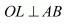and join OA.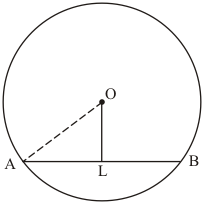Since, the perpendicular from the centre of a circle to a chord bisects the chord.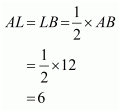Now in  we have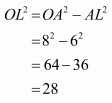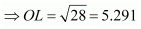Hence the distance of chord from the centre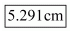.

Question 2 : Find the length of a chord which is at a distance of 5 cm from the centre of a circle of radius 10 cm.

Given that OA = 10 cm and OL = 5 cm, we have to find the length of chord AB.
Let AB be a chord of a circle with centre O and radius 10 cm such that AO = 10 cm
We drawand join OA.Since, the perpendiculars from the centre of a circle to a chord bisect the chord.
Now in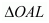we have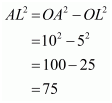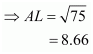Hence the length of chord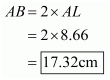Question 3 : Find the length of a chord which is at a distance of 4 cm from the centre of the circle of radius 6 cm.

Given that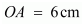and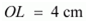, find the length of chord AB.
Let AB be a chord of a circle with centre O and radius 6 cm such that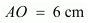We draw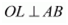and join OA.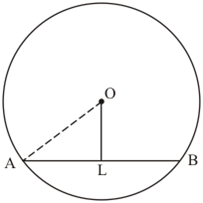Since, the perpendicular from the centre of a circle to a chord bisects the chord.
Now inwe have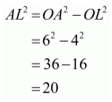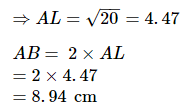Hence the length of the chord is 8.94 cm.

Question 4 : Two chords AB, CD of lengths 5 cm, 11 cm respectively of a circle are parallel, If the distance between AB and CD is 3 cm, find the radius of the circle.

Answer 4 : Let AB and CD be two parallel chord of the circle with centre O such that AB = 5 cm and CD = 11 cm. let the radius of the circle be  cm.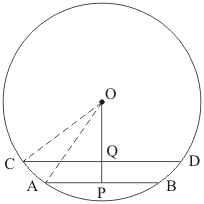Draw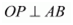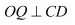and  as well as point O, Q and P are collinear.
Clearly, PQ = 3 cm
Let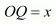then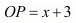In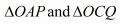we have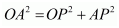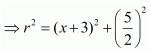…… (2)
From (1) and (2) we get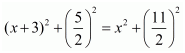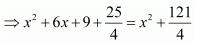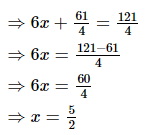Putting the value of x in (2) we get,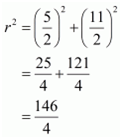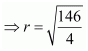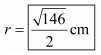Question 5 : Give a method to find the centre of a given circle.

Let A, B and C are three distinct points on a circle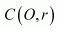.
Now join AB and BC and draw their perpendicular bisectors.
The point of intersection of the perpendicular bisectors is the centre of given circle.
Hence O is the centre of circle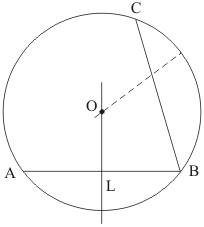.

Question 6 : Prove that the line joining the mid-point of a chord to the centre of the circle passes through the mid-point of the corresponding minor arc.

Let P is the mid point of chord AB of circle C(O, r) then according to question, line OQ passes through the point P.
Then prove that OQ bisect the arc AB.
Join OA and OB.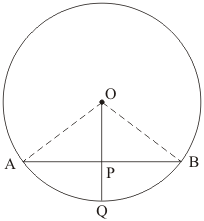In △AOP and △BOP△AOP and △BOP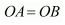(Radii of the same circle)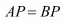(P is the mid point of chord AB)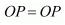(Common)
Therefore,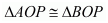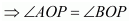(by cpct)
Thus
Arc AQ = arc BQ
Therefore,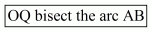Hence Proved.

Question 7 : Prove that a diameter of a circle which bisects a chord of the circle also bisects the angle subtended by the chord at the centre of the circle.

Let MN is the diameter and chord AB of circle C(O, r) then according to the question
AP = BP.
Then we have to prove that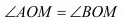.
Join OA and OB.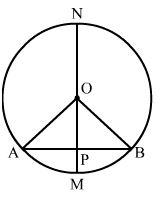In ΔAOP and ΔBOP(Radii of the same circle)
AP = BP         (P is the mid point of chord AB)
OP = OP         (Common)
Therefore,(by cpct)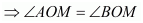Hence, proved.

Question 8 : Prove that two different circles cannot intersect each other at more than two points.

We have to prove that two different circles cannot intersect each other at more than two points.
Let the two circles intersect in three points A, B and C.
Then as we know that these three points A, B and C are non-collinear. So, a unique circle passes through these three points.
This is a contradiction to the fact that two given circles are passing through A, B, C.
Hence, two circles cannot intersect each other at more than two points.
Hence, proved.

Question 9 : A line segment AB is of length 5cm. Draw a circle of radius 4 cm passing through A and B. Can you draw a circle of radius 2 cm passing through A and B? Give reason in support of your answer.

Given that a line AB = 5 cm, one circle having radius of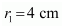which is passing through point A and B and other circle of radius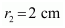.
As we know that the largest chord of any circle is equal to the diameter of that circle.
So,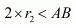There is no possibility to draw a circle whose diameter is smaller than the length of the chord.

Question 10 : An equilateral triangle of side 9 cm is inscribed in a circle. Find the radius of the circle.

Answer 10 : Let ABC be an equilateral triangle of side 9 cm and let AD be one of its medians. Let G be the centroid of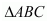. Then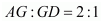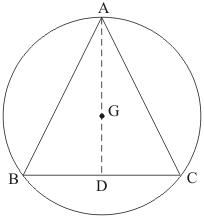We know that in an equilateral triangle centroid coincides with the circumcentre. Therefore, G is the centre of the circumcircle with circumradius GA.
As per theorem, G is the centre and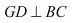. Therefore,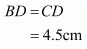In  we have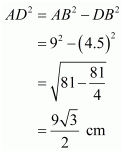Therefore radius AG =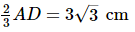Todays Deals### RD Chapter 16- Circles Ex-16.2 Contributorskrishan

Name:
Email:

# Latest News# 9000 interview questions in different categories